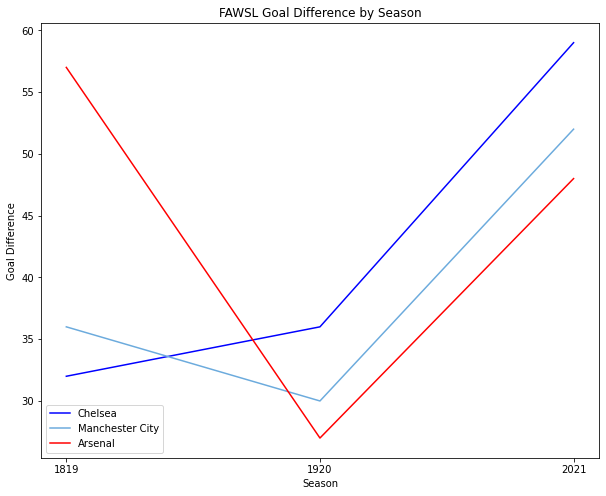# competitiveness-gd-xgd¶

Data visualisatons looking at goal difference in Europe's top 5 leagues, using goal difference variance to measure a league's competitiveness. Data from FBref covers seasons 2017/18, 2018/19, 2019/20, 2020/21 for Ligue 1, Serie A, La Liga, Premier League, Bundesliga, and FA Women's Super League.

If a club beats a club 1-0, they earn 3 points. If a club thrashes a club 10-0, they still only earn 3 points. Looking at the the points alone does not reveal the full extent to which a club is dominant or falling behind. Looking at goal difference accrued across a season reveals much more about which teams are the most dominant. A club accumulating a large amount of goal difference indicates that they do not experience tough opposition during the season. With this in mind, we can use the variance in GD between clubs to ascertain which leagues are the most competitive.

In :
import numpy as np
import pandas as pd
import os
import matplotlib.pyplot as plt
import ipywidgets as widgets
from IPython.display import display
from scipy import stats
import csv
from matplotlib.pyplot import figure

#read csv files --- data from fbref
epl_df = pd.read_csv (r'epl.csv')
seriea_df = pd.read_csv (r'seriea.csv')
ligue1_df = pd.read_csv (r'ligue1.csv')
bundesliga_df = pd.read_csv (r'bundesliga.csv')
laliga_df = pd.read_csv (r'laliga.csv')
fawsl_df = pd.read_csv (r'fawsl.csv')


### xGD & GD Correlation¶

First, there is a decision to make. When analysing GD, we can either look at actual goals scored and conceded, or expected goals scored and conceded. We first need to establish if these two metrics, xGD and GD, correlate with each other. If there is a very strong link between a club's xGD and GD, it should not matter too much which we use because they will mirror each other closely. When assessing the correlation between these two metrics in the top 5 leagues, as done below, we find a correlation of 0.9+ in each league. Because of this, GD will be used as our metric of choice throughout.

In :
x=[]
y=[]

x = epl_df['xGD'].tolist()
y = epl_df['GD'].tolist()

slope, intercept, r, p, std_err = stats.linregress(x, y)

def myfunc(x):
return slope * x + intercept

mymodel = list(map(myfunc, x))
r = round(r, 3)
print("Premier League Correlation Coefficient:", r)

x=[]
y=[]

x = laliga_df['xGD'].tolist()
y = laliga_df['GD'].tolist()

slope, intercept, r, p, std_err = stats.linregress(x, y)

def myfunc(x):
return slope * x + intercept

mymodel = list(map(myfunc, x))
r = round(r, 3)
print("La Liga Correlation Coefficient:", r)

x=[]
y=[]

x = seriea_df['xGD'].tolist()
y = seriea_df['GD'].tolist()

slope, intercept, r, p, std_err = stats.linregress(x, y)

def myfunc(x):
return slope * x + intercept

mymodel = list(map(myfunc, x))
r = round(r, 3)
print("Serie A Correlation Coefficient:", r)

x=[]
y=[]

x = bundesliga_df['xGD'].tolist()
y = bundesliga_df['GD'].tolist()

slope, intercept, r, p, std_err = stats.linregress(x, y)

def myfunc(x):
return slope * x + intercept

mymodel = list(map(myfunc, x))

r = round(r, 3)
print("Bundesliga Correlation Coefficient:", r)

x=[]
y=[]

x = ligue1_df['xGD'].tolist()
y = ligue1_df['GD'].tolist()

slope, intercept, r, p, std_err = stats.linregress(x, y)

def myfunc(x):
return slope * x + intercept

mymodel = list(map(myfunc, x))
r = round(r, 3)
print("Ligue 1 Correlation Coefficient:", r)

Premier League Correlation Coefficient: 0.94
La Liga Correlation Coefficient: 0.908
Serie A Correlation Coefficient: 0.934
Bundesliga Correlation Coefficient: 0.94
Ligue 1 Correlation Coefficient: 0.907


### Expected Goals Under/Over Performance¶

Although xGD and GD closely mirror eachother, the below clubs over or underperformed their expected GD more than any others. I have included this in case its of interest. There is strong disagreement over whether underperformance is down to poor finishing or bad luck, so I won't attempt to conclude too much from this information.

In :
top_5 = [epl_df, seriea_df, laliga_df, bundesliga_df, ligue1_df]

top_5_df = pd.concat(top_5)
top_5_df['xgg'] = top_5_df.GF - top_5_df.xG

print(top_5_df.sort_values(by=['xgg']))

    Season          Squad  GF  GA  GD  Pts    xG   xGA   xGD   xgg
70    1718       Sassuolo  29  59 -30   43  49.4  49.3   0.1 -20.4
75    1718           Caen  27  52 -25   38  42.2  48.7  -6.6 -15.2
17    2021         Fulham  27  53 -26   28  41.3  52.9 -11.7 -14.3
46    1819           Nice  30  35  -5   56  44.2  49.0  -4.8 -14.2
78    1718     Las Palmas  24  74 -50   22  38.0  65.2 -27.3 -14.0
..     ...            ...  ..  ..  ..  ...   ...   ...   ...   ...
0     2021  Bayern Munich  99  44  55   78  75.8  41.0  34.8  23.2
61    1718         Monaco  85  45  40   80  61.5  47.0  14.5  23.5
19    1920       Dortmund  84  41  43   69  59.2  39.4  19.8  24.8
64    1718          Lazio  89  49  40   72  63.5  44.4  19.0  25.5
60    1718       Juventus  86  24  62   95  59.8  28.7  31.0  26.2

[392 rows x 10 columns]


### Big 5 Leagues: Variance by Season¶

The below bar chart summarises the statistical variance for each of the big 5 leagues. A higher variance indicates a greater difference in the abilities of the clubs in the league. A low variance indicates that the league is competitive and that there are fewer teams conceding/scoring an outlandishly high number of goals.

The biggest lesson from the chart is that variance varies! No league sticks out in particular for having consistently high or low variance.

2019/20 Ligue 1 has the lowest variance, whilst 2018/19 Premier League has the highest. Perhaps we would argue that this means the Premier League was uncompetitive in 2018/19, but fans will remember that the league was decided by one point between frontrunners City and Liverpool: a true title race with exciting competition between two giants. Contrariwise, the 2019/20 Ligue 1 season, which statistically was the most competitive, was a walk in the park for PSG. My theory for this is that competition was tight in Ligue 1 for places 2-20, whilst in the Premier League, Liverpool and City racked up a much higher GD than the other 18 clubs.

In :
epl_2021_df = epl_df[(epl_df.Season == 2021)]
epl_2021_var = epl_2021_df.loc[:,"GD"].var()

epl_1920_df = epl_df[(epl_df.Season == 1920)]
epl_1920_var = epl_1920_df.loc[:,"GD"].var()

epl_1819_df = epl_df[(epl_df.Season == 1819)]
epl_1819_var = epl_1819_df.loc[:,"GD"].var()

epl_1718_df = epl_df[(epl_df.Season == 1718)]
epl_1718_var = epl_1718_df.loc[:,"GD"].var()

epl_var = [epl_1718_var, epl_1819_var, epl_1920_var, epl_2021_var]

seriea_2021_df = seriea_df[(seriea_df.Season == 2021)]
seriea_2021_var = seriea_2021_df.loc[:,"GD"].var()

seriea_1920_df = seriea_df[(seriea_df.Season == 1920)]
seriea_1920_var = seriea_1920_df.loc[:,"GD"].var()

seriea_1819_df = seriea_df[(seriea_df.Season == 1819)]
seriea_1819_var = seriea_1819_df.loc[:,"GD"].var()

seriea_1718_df = seriea_df[(seriea_df.Season == 1718)]
seriea_1718_var = seriea_1718_df.loc[:,"GD"].var()

seriea_var = [seriea_1718_var, seriea_1819_var, seriea_1920_var, seriea_2021_var]

laliga_2021_df = laliga_df[(laliga_df.Season == 2021)]
laliga_2021_var = laliga_2021_df.loc[:,"GD"].var()

laliga_1920_df = laliga_df[(laliga_df.Season == 1920)]
laliga_1920_var = laliga_1920_df.loc[:,"GD"].var()

laliga_1819_df = laliga_df[(laliga_df.Season == 1819)]
laliga_1819_var = laliga_1819_df.loc[:,"GD"].var()

laliga_1718_df = laliga_df[(laliga_df.Season == 1718)]
laliga_1718_var = laliga_1718_df.loc[:,"GD"].var()

laliga_var = [laliga_1718_var, laliga_1819_var, laliga_1920_var, laliga_2021_var]

bundesliga_2021_df = bundesliga_df[(bundesliga_df.Season == 2021)]
bundesliga_2021_var = bundesliga_2021_df.loc[:,"GD"].var()

bundesliga_1920_df = bundesliga_df[(bundesliga_df.Season == 1920)]
bundesliga_1920_var = bundesliga_1920_df.loc[:,"GD"].var()

bundesliga_1819_df = bundesliga_df[(bundesliga_df.Season == 1819)]
bundesliga_1819_var = bundesliga_1819_df.loc[:,"GD"].var()

bundesliga_1718_df = bundesliga_df[(bundesliga_df.Season == 1718)]
bundesliga_1718_var = bundesliga_1718_df.loc[:,"GD"].var()

bundesliga_var = [bundesliga_1718_var, bundesliga_1819_var, bundesliga_1920_var, bundesliga_2021_var]

ligue1_2021_df = ligue1_df[(ligue1_df.Season == 2021)]
ligue1_2021_var = ligue1_2021_df.loc[:,"GD"].var()

ligue1_1920_df = ligue1_df[(ligue1_df.Season == 1920)]
ligue1_1920_var = ligue1_1920_df.loc[:,"GD"].var()

ligue1_1819_df = ligue1_df[(ligue1_df.Season == 1819)]
ligue1_1819_var = ligue1_1819_df.loc[:,"GD"].var()

ligue1_1718_df = ligue1_df[(ligue1_df.Season == 1718)]
ligue1_1718_var = ligue1_1718_df.loc[:,"GD"].var()

ligue1_var = [ligue1_1718_var, ligue1_1819_var, ligue1_1920_var, ligue1_2021_var]

barWidth = 0.1
r1 = np.arange(len(epl_var))
r2 = [x + barWidth for x in r1]
r3 = [x + barWidth for x in r2]
r4 = [x + barWidth for x in r3]
r5 = [x + barWidth for x in r4]

plt.figure(figsize=(10,8))
plt.bar(r1, epl_var, color='cyan', width=barWidth, edgecolor='white', label='Premier League')
plt.bar(r2, seriea_var, color='magenta', width=barWidth, edgecolor='white', label='Serie A')
plt.bar(r3, laliga_var, color='yellow', width=barWidth, edgecolor='white', label='La Liga')
plt.bar(r4, bundesliga_var, color='gray', width=barWidth, edgecolor='white', label='Bundesliga')
plt.bar(r5, ligue1_var, color='#39FF14', width=barWidth, edgecolor='white', label='Ligue 1')
plt.xlabel('Season')
plt.ylabel('GD Variance')
plt.title('GD Variance by Season')
plt.xticks(np.arange(4), ['2017/18', '2018/19', '2019/20', '2020/21'], rotation=90)

plt.legend()
plt.savefig('gdvar.svg')
plt.show()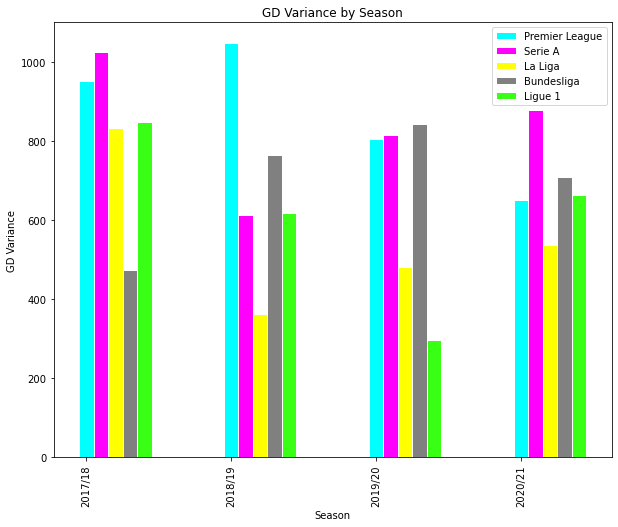### EPL & FAWSL: Variance¶

By taking a second to compare the variance of the Premier League with the variance of England's top tier women's league, the FAWSL, we can objectively test the hypothesis that women's football is less competitive.

In fact, when looking at this metric, the FAWSL had lower GD variance in 2018/19 and 2019/20, indicating a higher level of competitiveness. This changes drastically in 2020/21, where the FAWSL's variance is off the charts. This change is reflected in data studied later on.

In :
epl_var_1 = [epl_1819_var, epl_1920_var, epl_2021_var]

fawsl_2021_df = fawsl_df[(fawsl_df.Season == 2021)]
fawsl_2021_var = fawsl_2021_df.loc[:,"GD"].var()

fawsl_1920_df = fawsl_df[(fawsl_df.Season == 1920)]
fawsl_1920_var = fawsl_1920_df.loc[:,"GD"].var()

fawsl_1819_df = fawsl_df[(fawsl_df.Season == 1819)]
fawsl_1819_var = fawsl_1819_df.loc[:,"GD"].var()

fawsl_var = [fawsl_1819_var, fawsl_1920_var, fawsl_2021_var]

barWidth = 0.2
r1 = np.arange(len(epl_var_1))
r2 = [x + barWidth for x in r1]

plt.bar(r1, epl_var_1, color='cyan', width=barWidth, edgecolor='white', label='Premier League')
plt.bar(r2, fawsl_var, color='magenta', width=barWidth, edgecolor='white', label='FA Womens Super League')
plt.xlabel('Season')
plt.ylabel('GD Variance')
plt.title('GD Variance by Season')
plt.xticks(np.arange(3), ['2018/19', '2019/20', '2020/21'], rotation=90)

plt.legend()
plt.savefig('gdvarwoso.svg')
plt.show()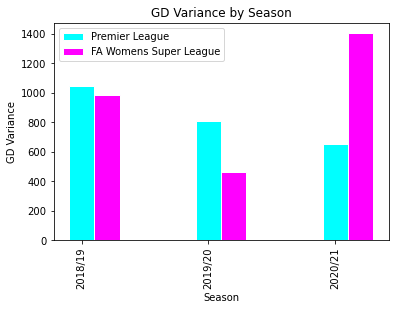### Goal Difference Distributions by Season¶

As was clear when discussing the 2019/20 Ligue 1 season, variance alone does not tell the full story of a league's competitiveness. To get a better picture, it is useful to look at how GD is distributed across the league. Are there some outliers at the top or bottom? Is there a lot of variation in the middle of the pack (the interquartile range)? What is the difference between the club with the highest GD and the club with the lowest?

The below notes reflect my insights from these charts.

#### Premier League¶

• The 18/19 season, with the highest variance, looks as expected. The two outliers, Manchester City and Liverpool, sit much higher than the rest of the league. The interquartile range (middle 50% of clubs) is small (between +20 and -20), but the club with the lowest GD had the lowest GD across all 4 seasons. This, combined with the two outliers, might be why this season has the highest variance of all
• The 17/18 season has the highest GD and the widest interquartile range, indicating high variance
• Variance is decreasing, indicating that the league is becoming more competitive. In particular, the median has improved, reaching above 0 GD for the first time last season
• Since 2019/20, no club has had over 60 GD in a season

#### La Liga¶

• Until last season, each La Liga season had at least 2 outliers with very high GD, indicating an elite within the league (no guesses for which clubs these outliers are!)
• Season 17/18 had a small interquartile range, indicating that the middling clubs were all of similar ability, but as well as three outliers at the top, the bottom club is also a statistical outlier, indicating a very large spread of GD
• The median GD has been below 0 every season, but the lowest ranked club now has much better GD than in previous seasons, indicating improved standards in the league

#### Serie A¶

• Serie A's box plots look very similar from season to season, with the main change being that the maximum value has declined, indicating that the presence of a frontrunner has declined over time
• Serie A in 20/21 looks more competitive than it was in 17/18
• The lowest GD club each season has a GD of almost -60, much lower than in the other leagues. This indicates that there is almost always a weak bottom placed club in the league

#### Bundesliga¶

• Bundesliga's upper limit is comparatively higher than its lower limit, indicating that the league is dominated by one club, but that there are no particularly weak clubs in the league
• The presence of one dominant club is reflected in the presence of outliers
• The 2017/18 interquartile range is particularly small, indicating strong mid table competitiveness

#### Ligue 1¶

• Every season has an outlier at the top, indicating a lack of competition for the top spot
• The box plot in 2019/20 is very short, asides from the top and bottom outliers, suggesting a very competitive fight for places 2-19
• 20/21 saw the worst GD for a club so far, potentially indicating a fall in standards

#### FAWSL¶

• The pattern here reflects the observations made from the variance values for this league. A highly competitive 2019/20 season and a much less competitive 20/21
• Notably there are no statistical outliers on the chart, indicating that, whilst there is a lack of competitiveness, it is not being driven by one especially strong team, but a more general inequality between the strong and the weak sides
In :
epl_df.boxplot(column='GD',
by='Season',
color='cyan',
rot=90)
plt.ylim([-60, 80])
plt.title("Premier League Goal Difference by Season")
plt.suptitle('')
plt.savefig('eplbox.svg')

laliga_df.boxplot(column='GD',
by='Season',
color='yellow',
rot=90)
plt.ylim([-60, 80])
plt.title("La Liga Goal Difference by Season")
plt.suptitle('')
plt.savefig('laligabox.svg')

seriea_df.boxplot(column='GD',
by='Season',
color='magenta',
rot=90)
plt.ylim([-60, 80])
plt.title("Serie A Goal Difference by Season")
plt.suptitle('')
plt.savefig('serieabox.svg')

bundesliga_df.boxplot(column='GD',
by='Season',
color='gray',
rot=90)
plt.ylim([-60, 80])
plt.title("Bundesliga Goal Difference by Season")
plt.suptitle('')
plt.savefig('bundesligabox.svg')

ligue1_df.boxplot(column='GD',
by='Season',
color='#39FF14',
rot=90)
plt.ylim([-60, 80])
plt.title("Ligue 1 Goal Difference by Season")
plt.suptitle('')
plt.savefig('ligue1box.svg')

fawsl_df.boxplot(column='GD',
by='Season',
color='purple',
rot=90)
plt.ylim([-60, 80])
plt.title("FAWSL Goal Difference by Season")
plt.suptitle('')
plt.savefig('fawslbox.svg')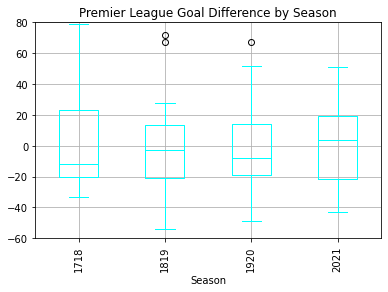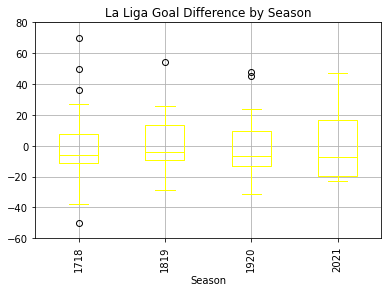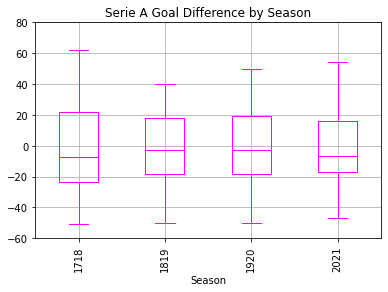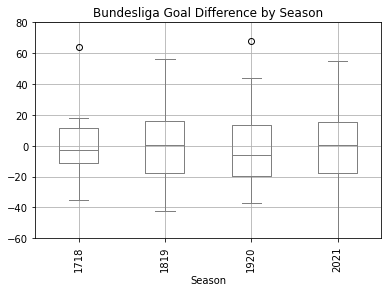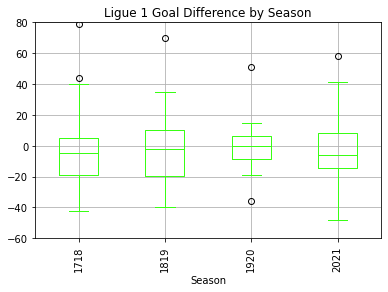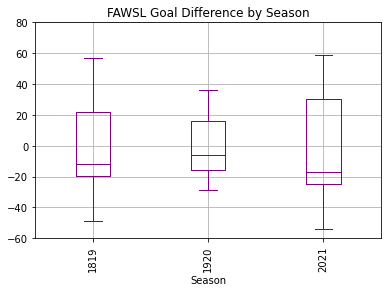### Goal Difference Over Time by Club¶

The below graphs chart the GD performance of all big5 league clubs that qualified for the Champions League through their league finish since 2017/18. The purpose of these charts is to illustrate how competitive the top end of each league is. Are there lots of clubs all performing at a high level? Does the same club register the highest GD each season? Are there any underdog stories?

The below comments are some of the observations I noticed from studying the graphs.

#### Premier League:¶

• City dominance is clear and obvious, but their GD has been declining
• Liverpool's "best" season was 18/19, where they narrowly missed out on the title, rather than the 19/20 winning season
• The 18/19 season is the season with the highest variance, it stands out on the graph, with Liverpool and City registering a GD number much higher than anyone else
• 20/21, where key players were injured, was a real low point for Liverpool
• Chelsea and Spurs have had very similar GD for the past four years

#### La Liga:¶

• Clear dominance of Barcelona, Atletico, and Real.
• Surprising to see that Barcelona has recorded consistently higher GD
• Sevilla overtaking Valencia as the number four team
• Real Madrid had a real dip in their first season without CR7, but recovered quickly
• The big three clubs were the only ones with positive GD each year

#### Serie A:¶

• Performance of the top clubs by GD varies a lot each season
• Juventus decline is clearly visible, as is the rise of Inter Milan, who narrowly missed out on 19/20 and secured the 20/21 league
• Atalanta come out of it looking strong
• Signs of improvement for AC Milan and decline for AS Roma

#### Bundesliga:¶

• Seems to be the most predictable league - the top clubs perform very similarly for GD each season
• Schalke 04 have declined dramatically, culminating in their relegation last season
• Bayern Munich are untouchable at the top
• Competition is extremely close between Borussia Dortmund and RB Leipzig for second best

#### Ligue 1:¶

• The fight for best of the rest is tight, but PSG's dominance is considerable, even last season when Lille won the league
• Lyon make the strongest argument for being second best

#### FAWSL:¶

• Arsenal were dominant in 2018/19, but have been third best since
• The spike in GD for the top three indicates that the league might be becoming less competitive
• in 19/20 and 20/21, the top three performed very similarly to each other
In :
mancity_df = epl_df[(epl_df.Squad == "Manchester City")]
manunited_df = epl_df[(epl_df.Squad == "Manchester Utd")]
liverpool_df = epl_df[(epl_df.Squad == "Liverpool")]
chelsea_df = epl_df[(epl_df.Squad == "Chelsea")]
spurs_df = epl_df[(epl_df.Squad == "Tottenham")]
plt.figure(figsize=(10,8))
plt.plot(mancity_df.Season, mancity_df.GD, color = "#6CABDD", label = "Manchester City")
plt.plot(manunited_df.Season, manunited_df.GD, color = "Green", label = "Manchester United")
plt.plot(spurs_df.Season, spurs_df.GD, color = "Grey", label = "Tottenham Hotspur")
plt.plot(chelsea_df.Season, chelsea_df.GD, color = "blue", label = "Chelsea")
plt.plot(liverpool_df.Season, liverpool_df.GD, color = "red", label = "Liverpool")
plt.xlabel('Season')
plt.xticks([1718, 1819, 1920, 2021])
plt.ylabel('Goal Difference')
plt.title('Premier League Goal Difference by Season')
plt.legend()
plt.savefig('eplgdline.svg')
plt.show()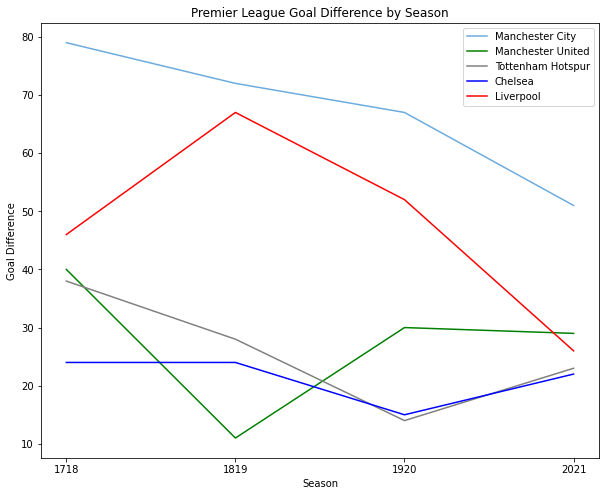In :
barca_df = laliga_df[(laliga_df.Squad == "Barcelona")]
sevilla_df = laliga_df[(laliga_df.Squad == "Sevilla")]
valencia_df = laliga_df[(laliga_df.Squad == "Valencia")]
plt.figure(figsize=(10,8))
plt.plot(barca_df.Season, barca_df.GD, color = "blue", label = "Barcelona")
plt.plot(atleti_df.Season, atleti_df.GD, color = "red", label = "Atlético Madrid")
plt.plot(real_df.Season, real_df.GD, color = "Gold", label = "Real Madrid")
plt.plot(sevilla_df.Season, sevilla_df.GD, color = "grey", label = "Sevilla")
plt.plot(valencia_df.Season, valencia_df.GD, color = "orange", label = "Valencia")
plt.xlabel('Season')
plt.xticks([1718, 1819, 1920, 2021])
plt.ylabel('Goal Difference')
plt.title('La Liga Goal Difference by Season')
plt.legend()
plt.savefig('laligagdline.svg')
plt.show()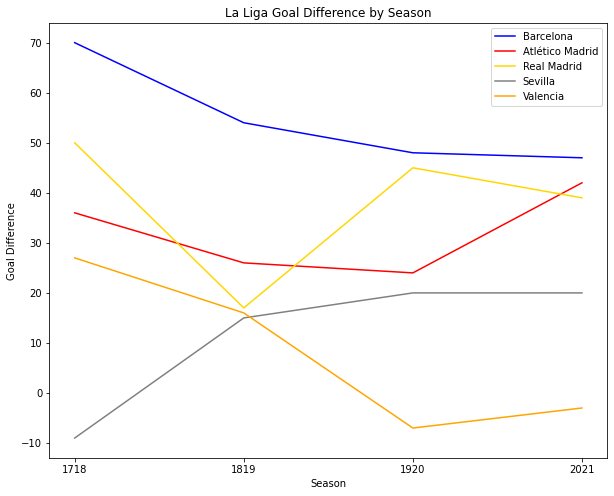In :
juventus_df = seriea_df[(seriea_df.Squad == "Juventus")]
inter_df = seriea_df[(seriea_df.Squad == "Inter")]
napoli_df = seriea_df[(seriea_df.Squad == "Napoli")]
lazio_df = seriea_df[(seriea_df.Squad == "Lazio")]
atalanta_df = seriea_df[(seriea_df.Squad == "Atalanta")]
milan_df = seriea_df[(seriea_df.Squad == "Milan")]
roma_df = seriea_df[(seriea_df.Squad == "Roma")]

plt.figure(figsize=(10,8))
plt.plot(juventus_df.Season, juventus_df.GD, color = "black", label = "Juventus")
plt.plot(inter_df.Season, inter_df.GD, color = "blue", label = "Inter")
plt.plot(napoli_df.Season, napoli_df.GD, color = "#12A0D7", label = "Napoli")
plt.plot(lazio_df.Season, lazio_df.GD, color = "#87D8F7", label = "Lazio")
plt.plot(atalanta_df.Season, atalanta_df.GD, color = "silver", label = "Atalanta")
plt.plot(milan_df.Season, milan_df.GD, color = "red", label = "Milan")
plt.plot(roma_df.Season, roma_df.GD, color = "#F0BC42", label = "Roma")
plt.xlabel('Season')
plt.xticks([1718, 1819, 1920, 2021])
plt.ylabel('Goal Difference')
plt.title('Serie A Goal Difference by Season')
plt.legend()
plt.savefig('serieagdline.svg')
plt.show()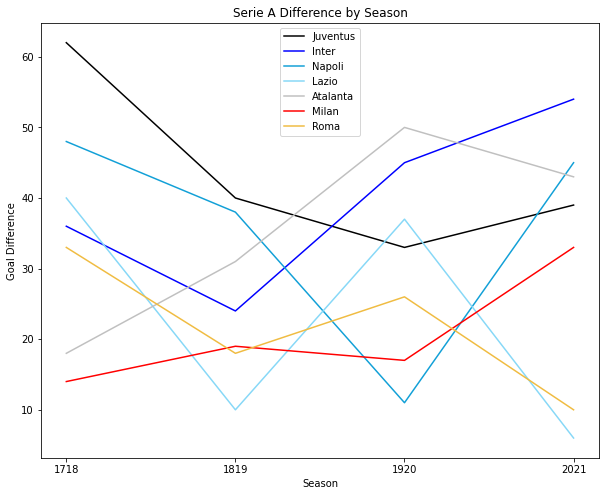In :
bmunich_df = bundesliga_df[(bundesliga_df.Squad == "Bayern Munich")]
bvb_df = bundesliga_df[(bundesliga_df.Squad == "Dortmund")]
rbl_df = bundesliga_df[(bundesliga_df.Squad == "RB Leipzig")]
wolfs_df = bundesliga_df[(bundesliga_df.Squad == "Wolfsburg")]
hoff_df = bundesliga_df[(bundesliga_df.Squad == "Hoffenheim")]
leverk_df = bundesliga_df[(bundesliga_df.Squad == "RLeverkusen")]
s04_df = bundesliga_df[(bundesliga_df.Squad == "Schalke 04")]

plt.figure(figsize=(10,8))
plt.plot(bmunich_df.Season, bmunich_df.GD, color = "red", label = "Bayern Munich")
plt.plot(bvb_df.Season, bvb_df.GD, color = "yellow", label = "Borussia Dortmund")
plt.plot(rbl_df.Season, rbl_df.GD, color = "gold", label = "RB Leipzig")
plt.plot(wolfs_df.Season, wolfs_df.GD, color = "#39FF14", label = "Wolfsburg")
plt.plot(leverk_df.Season, leverk_df.GD, color = "silver", label = "RLeverkusen")
plt.plot(s04_df.Season, s04_df.GD, color = "orange", label = "Schalke 04")
plt.plot(hoff_df.Season, hoff_df.GD, color = "blue", label = "Hoffenheim")
plt.xlabel('Season')
plt.xticks([1718, 1819, 1920, 2021])
plt.ylabel('Goal Difference')
plt.title('Bundesliga Goal Difference by Season')
plt.legend()
plt.savefig('bundesligagdline.svg')
plt.show()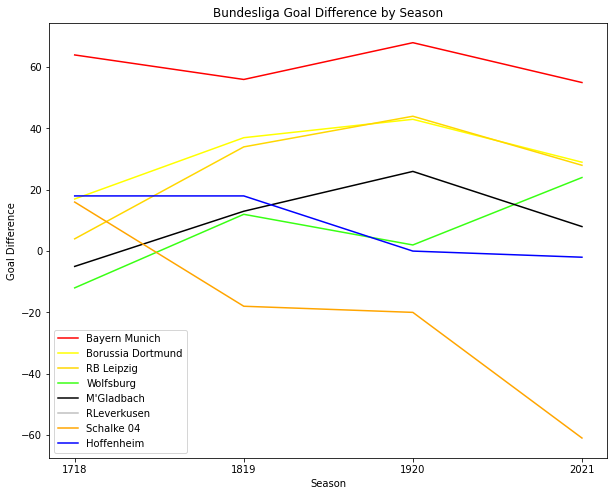In :
psg_df = ligue1_df[(ligue1_df.Squad == "Paris S-G")]
monaco_df = ligue1_df[(ligue1_df.Squad == "Monaco")]
lyon_df = ligue1_df[(ligue1_df.Squad == "Lyon")]
lille_df = ligue1_df[(ligue1_df.Squad == "Lille")]
marse_df = ligue1_df[(ligue1_df.Squad == "Marseille")]
rennes_df = ligue1_df[(ligue1_df.Squad == "Rennes")]

plt.figure(figsize=(10,8))
plt.plot(psg_df.Season, psg_df.GD, color = "blue", label = "PSG")
plt.plot(monaco_df.Season, monaco_df.GD, color = "red", label = "Monaco")
plt.plot(lyon_df.Season, lyon_df.GD, color = "gold", label = "Lyon")
plt.plot(lille_df.Season, lille_df.GD, color = "orange", label = "Lille")
plt.plot(marse_df.Season, marse_df.GD, color = "silver", label = "Marseille")
plt.plot(rennes_df.Season, rennes_df.GD, color = "purple", label = "Rennes")
plt.xlabel('Season')
plt.xticks([1718, 1819, 1920, 2021])
plt.ylabel('Goal Difference')
plt.title('Ligue 1 Goal Difference by Season')
plt.legend()
plt.savefig('ligue1gdline.svg')
plt.show()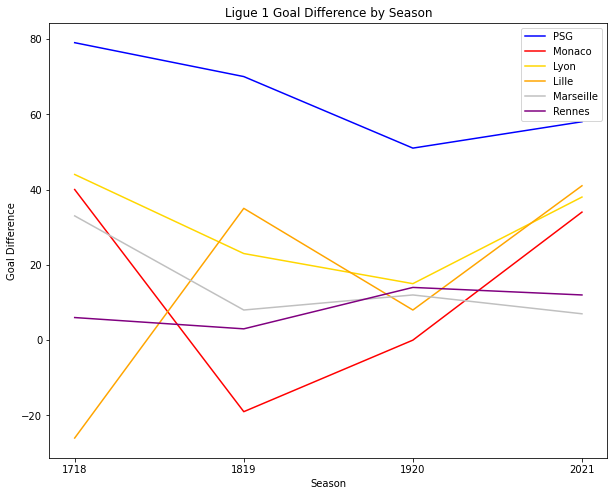In :
chelseaw_df = fawsl_df[(fawsl_df.Squad == "Chelsea")]
mancityw_df = fawsl_df[(fawsl_df.Squad == "Manchester City")]
arsenalw_df = fawsl_df[(fawsl_df.Squad == "Arsenal")]

plt.figure(figsize=(10,8))
plt.plot(chelseaw_df.Season, chelseaw_df.GD, color = "blue", label = "Chelsea")
plt.plot(mancityw_df.Season, mancityw_df.GD, color = "#6CABDD", label = "Manchester City")
plt.plot(arsenalw_df.Season, arsenalw_df.GD, color = "red", label = "Arsenal")
plt.xlabel('Season')
plt.xticks([1819, 1920, 2021])
plt.ylabel('Goal Difference')
plt.title('FAWSL Goal Difference by Season')
plt.legend()
plt.savefig('fawslgdline.svg')
plt.show()